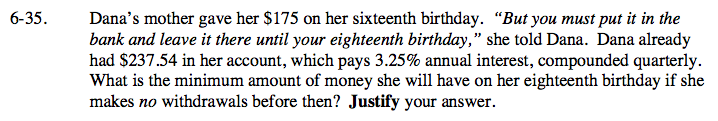### Home > A2C > Chapter Ch6 > Lesson 6.1.2 > Problem6-35

6-35.Notice that the interest is 3.25% annually, but it is compounded quarterly.

This means that you need to divide the percentage by 4, and multiply the number of compoundings by 4.

$\text{So, }\textit{A}(\textit{x})=(237.54 + 175)\cdot(1+\frac{0.0325}{4})^{4\textit{t}}$

\$440.13